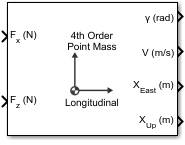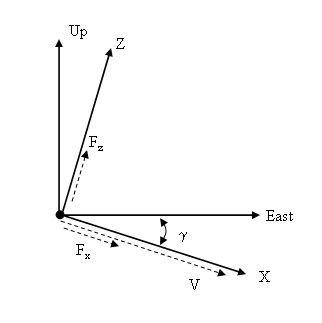# 4th Order Point Mass (Longitudinal)

Calculate fourth-order point mass

• Library:
• Aerospace Blockset / Equations of Motion / Point Mass

•## Description

The 4th Order Point Mass (Longitudinal) block performs the calculations for the translational motion of a single point mass or multiple point masses. For more information on the system for the translational motion of a single point mass or multiple mass, see Algorithms.

The 4th Order Point Mass (Longitudinal) block port labels change based on the input and output units selected from the Units list.

## Limitations

The flat Earth reference frame is considered inertial, an approximation that allows the forces due to the Earth's motion relative to the “fixed stars” to be neglected.

## Ports

### Input

expand all

Force in x-axis, specified as a scalar or array, in selected units.

Data Types: `double`

Force in z-axis, specified as a scalar or array, in selected units.

Data Types: `double`

### Output

expand all

Flight path angle, returned as a scalar or array, in radians.

Data Types: `double`

Airspeed, returned as a scalar or array, in selected units.

Data Types: `double`

Downrange or amount traveled east, returned as a scalar or array, in selected units.

Data Types: `double`

Altitude or amount traveled up, returned as a scalar or array, in selected units.

Data Types: `double`

## Parameters

expand all

Input and output units, specified as:

Units

Forces

Velocity

Position

Mass

`Metric (MKS)`

Newton

Meters per second

Meters

kilogram

```English (Velocity in ft/s)```

Pound

Feet per second

Feet

slugs

```English (Velocity in kts)```

Pound

Knots

Feet

slugs

#### Programmatic Use

 Block Parameter: `units` Type: character vector Values: ```'Metric (MKS)'``` | ```'English (Velocity in ft/s)'``` | ```'English (Velocity in kts)'``` Default: ```'Metric (MKS)'```

Initial flight path angle of the point mass(es), specified as a scalar or vector.

#### Programmatic Use

 Block Parameter: `gamma0` Type: character vector Values: scalar | vector Default: `'0'`

Initial airspeed of the point mass(es), specified as a scalar or vector.

#### Programmatic Use

 Block Parameter: `V0` Type: character vector Values: scalar | vector Default: `'100'`

Initial downrange of the point mass(es), specified as a scalar or vector.

#### Programmatic Use

 Block Parameter: `x0` Type: character vector Values: scalar | vector Default: `'0'`

Initial altitude of the point mass(es), specified as a scalar or vector.

#### Programmatic Use

 Block Parameter: `h0` Type: character vector Values: scalar | vector Default: `'0'`

Mass of the point mass(es), specified as a scalar or vector.

#### Programmatic Use

 Block Parameter: `mass0` Type: character vector Values: scalar | vector Default: `'1.0'`

## AlgorithmsThe translational motions of the point mass [XEastXUp]T are functions of airspeed (V) and flight path angle (γ),

`$\begin{array}{c}{F}_{x}=m\stackrel{˙}{V}\\ {F}_{z}=mV\stackrel{˙}{\gamma }\\ {\stackrel{˙}{X}}_{East}=V\mathrm{cos}\gamma \\ {\stackrel{˙}{X}}_{Up}=V\mathrm{sin}\gamma \end{array}$`

where the applied forces [FxFz]T are in a system defined as follows: x-axis is in the direction of vehicle velocity relative to air, z-axis is upward, and y-axis completes the right-handed frame. The mass of the body m is assumed constant.

## Extended Capabilities

### C/C++ Code GenerationGenerate C and C++ code using Simulink® Coder™.

Introduced before R2006a

## SupportGet trial now